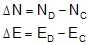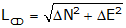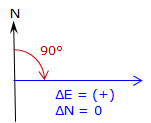## 2. Inverse Computation

An Inverse Computation determines the distance and direction between two coordinate pairs.Figure C-2 Inverse ComputationEquation C-2Equation C-3Equation C-4

Coordinate differences, Δ’s, are the to point minus the from point.

The algebraic sign on ß and the resulting direction depend on the quadrant of the line.Figure C-3 Quadrants

 Table C-1 Algebraic sign Direction Quadrant ΔN ΔE β Bearing Azimuth NE + + + N β E β SE - + - S |β| E 180°+β SW - - + S β W 180°+β NW + - - N |β| W 360°+β

A negative ß is a counter-clockwise angle.

These should all look familiar as they're the same equations from the Coordinates chapter of the Traverse Computations topic.

When ΔN = 0, Equation C-4 has no solution.Technically division by 0 is undefined, but actually the result of any number divided by 0 is infinity. Remember from the plot of the tangent function that tan(90°) = tan(270°) = infinity. So what does this mean?

In surveying terms when ΔN = 0 the entire line length is ΔE resulting in a due East (+) or West (-) line, Figure C-4.

 ΔE (+) → Az=90° ΔE (-) → Az=270°Figure C-4 When ΔN = 0